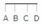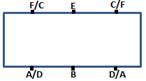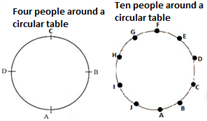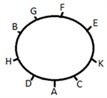# Logical Reasoning Questions

Being an integral section in the CAT exam, logical reasoning gives a hard time to many of the test-takers. The logical reasoning section demands the candidates to be extremely focused while solving. The logical reasoning section mainly includes questions related to arrangements (simple and complex). So, to help the CAT exam 2021 aspirants prepare properly, here is a brief explanation with an illustration of the logical reasoning section.

## Solving Logical Reasoning Questions: Tips

• LR problems usually contain several statements that serve as clues to solving the problem.
• It always helps if you have a great memory. The lesser number of times that you need to re-read the data, the more time you will get.
• The first step to solving an LR problem is to quickly jot down all the relevant information in a table or diagram. For example, if the problem involves seating arrangements at a round table then, always draw the table first and use the data in the question provided to arrange the objects.
• Once you have drawn the figure for the problem, you are free to think with an uncluttered mind.

### Logical Reasoning Questions and Solutions:

• Sequencing in a line:

A formal linear arrangement is one that emphasizes on lines and movement in an arrangement.

These types of questions could be based on “Seating Arrangement”.

Four people in line:Example 1:

Five people P, Q, R, S, and T are sitting in a row facing towards the north. P is two places away to the left of Q. R is two places away to the right of S.

T is not sitting at the extreme left as well as next to R. Who is sitting in the middle?

Solution:

As P is two places away to the left of Q and R is two places away to the right of S.

_ ?P ?S ?Q ?R , ?P ?S ?Q ?R _ , ?S ?P ?R ?Q _ , _ ?S ?P ?R ?Q

As T is not sitting at the extreme left as well as next to R. Out of four cases three cases are not possible.

Only S P R Q T is possible. So, R is sitting in the middle.

Example 2:

Six persons A, B, C, D, E, and F are sitting around a rectangular table facing each other ( three each side), not necessarily in the same order.

There is one person between C and F. E is neither adjacent to A nor D. F is opposite to A and D is opposite to C. Who is sitting opposite to E?

Solution:

Using the first condition – There is one person between C and F, i.e. C _ F or F _ C. So, there are only two conditions.

Using the second condition – E is neither adjacent to A nor D, i.e. C E F or F E C.

Using the third condition F is opposite A and D is opposite to C, the six persons can occupy the positions only as given in the diagram below.It is clear from the diagram, that B is opposite to E.

• Sequencing in a circle:

The arrangements we have considered so far are linear.

There are also arrangements in closed loops, called circular arrangements.Example:

A, B, C, D, E, F, G, H, and K are sitting around a circle facing the centre. F is fourth to the right of A who is third to the right of B.

K is fourth to the left of B and third to the right of D.C is third to the right of H. E is second to the left of G. Who is sitting immediate right of F?Solution:

Draw a circle and arrange the persons such that all the constraints are taken care of. G is sitting immediate to the right of F.

1.3.3 Selection Based Questions: These types of questions are based on positive groups and negative groups, based on the given conditions.

For example, the conditions may specify that two particular people should always be together or that two particular people should not be together.

For solving these type of questions, you can use flow charts and symbolic notations to deduce conclusions. In some questions, the Reverse Gear Approach is also a good method to eliminate options.

Example:

At least two boys out of A, B, C and D and at least two girls out of P, Q, R and S have to be chosen to form a group of five members.

Different teams are to be formed as per constraints below: –

(1)S and Q cannot be together.

(2)Neither A nor C can go with Q.

(3)Neither P nor S can go with C. Which of the following combinations is possible?